1. /
2. CBSE
3. /
4. Class 07
5. /
6. Mathematics
7. /
8. NCERT Solutions for Class...

# NCERT Solutions for Class 7 Maths Exercise 15.1### myCBSEguide App

Download the app to get CBSE Sample Papers 2023-24, NCERT Solutions (Revised), Most Important Questions, Previous Year Question Bank, Mock Tests, and Detailed Notes.

NCERT solutions for Maths Visualizing Solid Shapes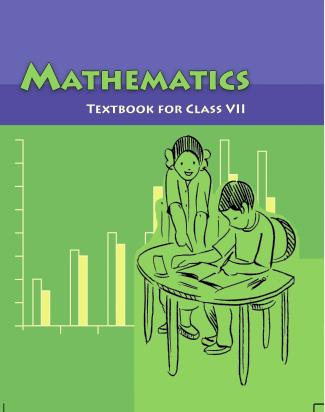## NCERT Solutions for Class 7 Maths Visualizing Solid Shapes

###### Questions 1. Identify the nets which can be used to make cubes (cut out copies of the nets and try it):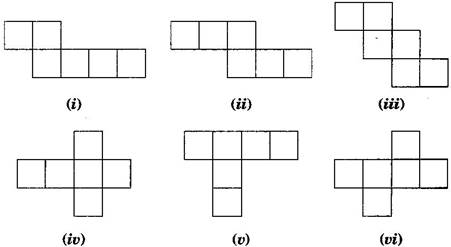Answers : Cube’s nets are (ii), (iii), (iv) and (vi).

NCERT Solutions for Class 7 Maths Exercise 15.1

###### Questions 2. Dice are cubes with dots on each face. Opposite faces of a die always have a total of seven dots on them.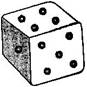Here are two nets to make dice (cubes); the numbers inserted in each square indicate the number of dots in that box.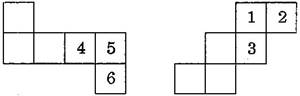Insert suitable numbers in the blanks, remembering that the number on the opposite faces should total to 7.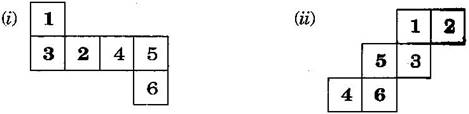Questions 3. Can this be a net for a die? Explain your answer.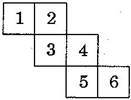Answers : No, this cannot be a net for a die.

Because one pair of opposite faces will have 1 and 4 on them and another pair of opposite faces will have 3 and 6 on them whose total is not equal to 7.

NCERT Solutions for Class 7 Maths Exercise 15.1

###### Questions 4. Here is an incomplete net for making a cube. Complete it in at least two different ways. Remember that a cube has six faces. How many faces are there in the net here? (Give two separate diagrams. If you like, you may use a squared sheet for easy manipulation.)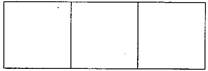Answers : There three faces are given: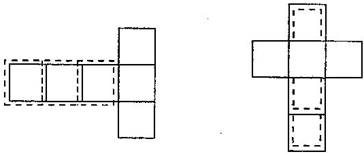NCERT Solutions for Class 7 Maths Exercise 15.1

###### Questions 5. Match the nets with appropriate solids: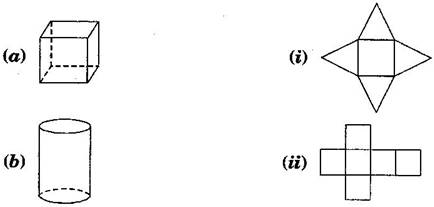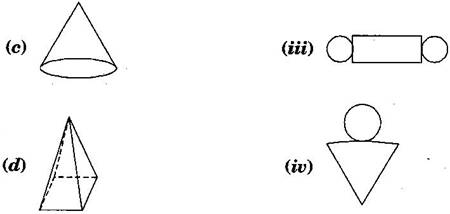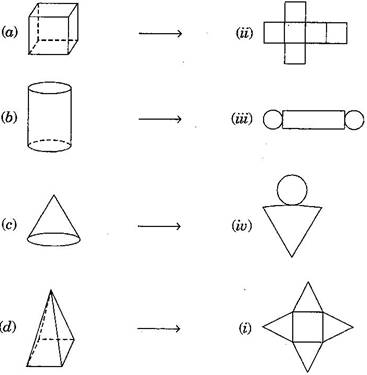## NCERT Solutions for Class 7 Maths Exercise 15.1

NCERT Solutions Class 7 Maths PDF (Download) Free from myCBSEguide app and myCBSEguide website. Ncert solution class 7 Maths includes text book solutions from Class 7 Maths Book . NCERT Solutions for CBSE Class 7 Maths have total 15 chapters. 7 Maths NCERT Solutions in PDF for free Download on our website. Ncert Maths class 7 solutions PDF and Maths ncert class 7 PDF solutions with latest modifications and as per the latest CBSE syllabus are only available in myCBSEguide.

## CBSE app for Students

To download NCERT Solutions for Class 7 Maths, Social Science Computer Science, Home Science, Hindi English, Maths Science do check myCBSEguide app or website. myCBSEguide provides sample papers with solution, test papers for chapter-wise practice, NCERT solutions, NCERT Exemplar solutions, quick revision notes for ready reference, CBSE guess papers and CBSE important question papers. Sample Paper all are made available through the best app for CBSE students and myCBSEguide website.### Test Generator

Create question paper PDF and online tests with your own name & logo in minutes.### myCBSEguide

Question Bank, Mock Tests, Exam Papers, NCERT Solutions, Sample Papers, Notes